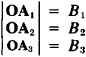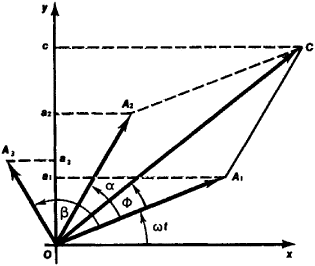Vector Diagram

Also found in: Dictionary, Thesaurus, Medical, Legal.

Vector Diagram

a graphical representation of the values of periodically varying quantities and the relationships between them by means of directed line segments called vectors.

Vector diagrams are widely used in electrical engineering, acoustics, optics, and other areas of science. The simple harmonic functions of one period, for example,

f1 = B1 sin ωt

f2 = B2 sin (α+ωt)

f3 = B3 sin (β+ωt)

can be represented graphically (see Figure 1) as projections on the Oy axis of vectors OA1OA2, and OA3 rotating with constant angular velocity ω, while OA2 and OA3 are turned by the angles α and β with reference to OA1. The length of the vectors corresponds to the amplitude of the oscillation:The sum or difference of two or more oscillations is indicated on a vector diagram as the geometric sum or difference of the vectors of the component oscillations, obtained according to the parallelogram rule, and the instantaneous value of the unknown quantity is determined by the projection of the vector sum on the Oy axis.Figure 1

For example, it is required to find the sum F of the oscillations fl with amplitude OA1 and f2, with amplitude OA2. By the geometric addition of vectors OA1 and OA2 on the vector diagram, we find that the amplitude of the summed oscillation F is equal to the length of vector OC = OA1 + OA2 and leads the oscillation f1 in phase by the angle φ

References in periodicals archive ?
Solution of the Vector Diagram for Resultant Force Vector
Our illustrative example of the vector diagram method to systematically synthesize advertising research verbatims shows only one of the infinite variety of possible resultant vectors.
There are very few hypotheses or assumptions to the vector diagram method.
All odd phase number vector diagrams have also one Feature--they can be divided into 2n symmetrical sectors in angle increments of [pi]/n , .

Site: Follow: Share:
Open / Close